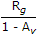# Electronics and Communication Engineering - Exam Questions Papers

36.

Which of the following statements is not correct for thermistors?

 A. They have negative temperature coefficient of resistance B. The sensing element is made of sintered ceramics, which are oxides of metals in the form of beads C. The variation of resistance with temperature is linear D. The resistance value at ambient temperature may range from 100 Ω to 100 kΩ

Explanation:

No answer description available for this question. Let us discuss.

37.

Which one of the following logic families can be operated using a supply voltage from 3 V to 15 V?

 A. TTL B. ECL C. PMOS D. CMOS

Explanation:

No answer description available for this question. Let us discuss.

38.

The circuit shown in the figure is used to charge the capacitor C alternately from two current sources as indicated. The switches S1 and S2 are mechanically coupled and connected as follow
For 2nT ≤ t < (2n + 1)T, (n = 0, 1, 2, ...) S1 to P1 and S2 to P2
For (2n + 1) T ≤ t < (2n + 2)T, (n = 0, 1, 2, ...) S1 to Q1 and S2 to Q2
Assume that the capacitor has zero initial charge. Given that u(t) is a unit step function, the voltage Vc(t) across the capacitor is given be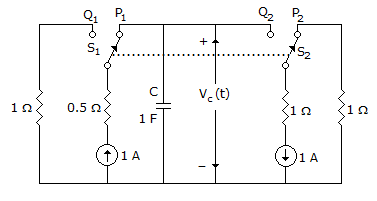A.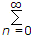(- 1)n tu(t - nT) B. u(t) + 2(-1)n u(t - nT) C. tu(t) + 2(-1)n (t - nT) u(t - nT) D.[0.5 - e-(t - 2nT) + 0.5e-(t - 2nT - T) ]

Explanation:

No answer description available for this question. Let us discuss.

39.

Which one of the following is a regulated power supply?

 A. IC 555 B. IC 844 C. IC 3080 D. IC 723

Explanation:

No answer description available for this question. Let us discuss.

40.

The input impedance of figure given below is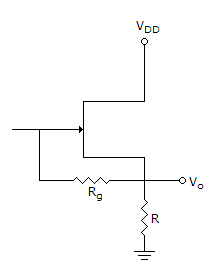A. Rg(1 + Av) B.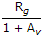C. Rg(1 - Av) D.